Next: Matrix element Up: Elements of the Fib Previous: Retrieve domain properties   Contents   Index

## Set-element

The set element assigns sets of values in succession to a number of variables. The variables apply everywhere in the subobject.

Syntax: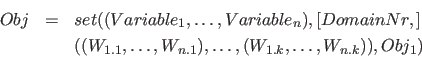Description of the elements:

•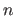: The number of elements of a set. The minimum number is one element. ()
•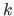: The number of sets of values, with which the variables will be set. The minimum number is one set. ()
•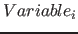: The variables, which the set element defines.
•: This is the number of the domain for the set-element. This information is optional, the default value is 0. If no set-domain with that number exist, the set-domain with the next smallest number is used. By using different, adapted for each set-element, domains, the memory requirements for storing in the compressed Fib format can be optimized.
•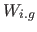with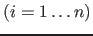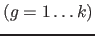: This are the to set values for the variables.
•: The vector with the sets of values to set.
•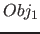: The subobject, for which the variablesare defined and which will be evalued for every variable assignment.

The variables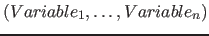are sequentially assigned to the individual sets of values of. Where the variablewill only be set to the values(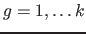). Thus there arebindings of variables, in which the variableis first set to the value of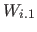, after this to, etc. . If an elementis a variable, thewill be set to the value of variable of.

Example:

•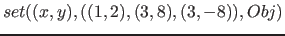; In this example the variablesand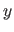will be set for the subobject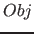sequentially to the values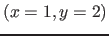,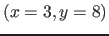and than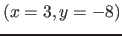.
•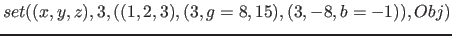; In this example the variables,andwill be set for the subobjectsequentially to the values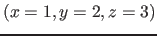,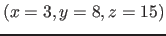and than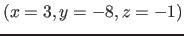. The domain for the set-element is the third set-domain.

Note: Not all dependencies of several variables can be easily represented by functions. Therefore, the set element offer the ability to assign multiple variables sequentially to sets of values.

It is conceivable, for example, to create a database object, which codes a character set ("font"). With the input parameters/variables of this database object the letter and the display position of the letters are assignable. However, it can not be assumed, that the input variables for the letters of a text are in a simple functional dependenc. With the set element the input variables can be easily assigned to the values for each letter of the text.Next: Matrix element Up: Elements of the Fib Previous: Retrieve domain properties   Contents   Index
Betti Österholz 2013-02-13